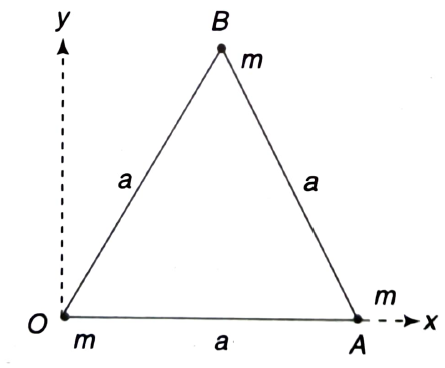Centre of mass(com)
Question

# Three particles each of mass m, are placed at the corners of an equilateral triangle of side a, as shown in Fig. The position vector of the centre of mass isModerate
Solution

## Refer to Fig. The (x, y) co-ordinates of the masses at 0, A and B respectively are $\left({\mathrm{x}}_{1}=0,{\mathrm{y}}_{1}=0\right),\left({\mathrm{x}}_{2}=\mathrm{a},{\mathrm{y}}_{2}=0\right),\left({\mathrm{x}}_{3}=\frac{\mathrm{a}}{2},{\mathrm{y}}_{3}=\frac{\mathrm{a}\sqrt{3}}{2}\right)$Therefore, the (x, y) co-ordinates of the centre of mass are  $\begin{array}{c}{\mathrm{x}}_{\mathrm{CM}}=\frac{\mathrm{m}×0+\mathrm{m}×\mathrm{a}+\mathrm{m}×\mathrm{a}/2}{\mathrm{m}+\mathrm{m}+\mathrm{m}}=\frac{\mathrm{a}}{2}\\ {\mathrm{y}}_{\mathrm{CM}}=\frac{\mathrm{m}×0+\mathrm{m}×0+\mathrm{m}×\mathrm{a}\sqrt{3}/2}{\mathrm{m}+\mathrm{m}+\mathrm{m}}\\ =\frac{\mathrm{a}}{2\sqrt{3}}\end{array}$$\therefore$Position vector of centre of mass is $\frac{\mathrm{a}}{2}\left(\stackrel{^}{\mathrm{i}}+\frac{\stackrel{^}{\mathrm{j}}}{\sqrt{3}}\right)$.

Get Instant Solutions